Plot likert scales as centered stacked bars.

plot_likert(
items,
groups = NULL,
groups.titles = "auto",
title = NULL,
legend.title = NULL,
legend.labels = NULL,
axis.titles = NULL,
axis.labels = NULL,
catcount = NULL,
cat.neutral = NULL,
sort.frq = NULL,
weight.by = NULL,
title.wtd.suffix = NULL,
wrap.title = 50,
wrap.labels = 30,
wrap.legend.title = 30,
wrap.legend.labels = 28,
geom.size = 0.6,
geom.colors = "BrBG",
cat.neutral.color = "grey70",
intercept.line.color = "grey50",
reverse.colors = FALSE,
values = "show",
show.n = TRUE,
show.legend = TRUE,
show.prc.sign = FALSE,
grid.range = 1,
grid.breaks = 0.2,
expand.grid = TRUE,
digits = 1,
reverse.scale = FALSE,
coord.flip = TRUE,
sort.groups = TRUE,
legend.pos = "bottom",
rel_heights = 1,
group.legend.options = list(nrow = NULL, byrow = TRUE),
cowplot.options = list(label_x = 0.01, hjust = 0, align = "v")
)

Arguments

items

Data frame, or a grouped data frame, with each column representing one item.

groups

(optional) Must be a vector of same length as ncol(items), where each item in this vector represents the group number of the related columns of items. See 'Examples'.

groups.titles

(optional, only used if groups are supplied) Titles for each factor group that will be used as table caption for each component-table. Must be a character vector of same length as length(unique(groups)). Default is "auto", which means that each table has a standard caption Component x. Use NULL to use names as supplied to groups and use FALSE to suppress table captions.

title

character vector, used as plot title. Depending on plot type and function, will be set automatically. If title = "", no title is printed. For effect-plots, may also be a character vector of length > 1, to define titles for each sub-plot or facet.

legend.title

character vector, used as title for the plot legend.

legend.labels

character vector with labels for the guide/legend.

axis.titles

character vector of length one or two, defining the title(s) for the x-axis and y-axis.

axis.labels

character vector with labels used as axis labels. Optional argument, since in most cases, axis labels are set automatically.

catcount

optional, amount of categories of items (e.g. "strongly disagree", "disagree", "agree" and "strongly agree" would be catcount = 4). Note that this argument only applies to "valid" answers, i.e. if you have an additional neutral category (see cat.neutral) like "don't know", this won't count for catcount (e.g. "strongly disagree", "disagree", "agree", "strongly agree" and neutral category "don't know" would still mean that catcount = 4). See 'Note'.

cat.neutral

If there's a neutral category (like "don't know" etc.), specify the index number (value) for this category. Else, set cat.neutral = NULL (default). The proportions of neutral category answers are plotted as grey bars on the left side of the figure.

sort.frq

Indicates whether the items of items should be ordered by total sum of positive or negative answers.

"pos.asc"

to order ascending by sum of positive answers

"pos.desc"

to order descending by sum of positive answers

"neg.asc"

"neg.desc"

NULL

(default) for no sorting

weight.by

Vector of weights that will be applied to weight all cases. Must be a vector of same length as the input vector. Default is NULL, so no weights are used.

title.wtd.suffix

Suffix (as string) for the title, if weight.by is specified, e.g. title.wtd.suffix=" (weighted)". Default is NULL, so title will not have a suffix when cases are weighted.

wrap.title

numeric, determines how many chars of the plot title are displayed in one line and when a line break is inserted.

wrap.labels

numeric, determines how many chars of the value, variable or axis labels are displayed in one line and when a line break is inserted.

wrap.legend.title

numeric, determines how many chars of the legend's title are displayed in one line and when a line break is inserted.

wrap.legend.labels

numeric, determines how many chars of the legend labels are displayed in one line and when a line break is inserted.

geom.size

size resp. width of the geoms (bar width, line thickness or point size, depending on plot type and function). Note that bar and bin widths mostly need smaller values than dot sizes.

geom.colors

user defined color for geoms. See 'Details' in plot_grpfrq.

cat.neutral.color

Color of the neutral category, if plotted (see cat.neutral).

intercept.line.color

Color of the vertical intercept line that divides positive and negative values.

reverse.colors

logical, if TRUE, the color scale from geom.colors will be reversed, so positive and negative values switch colors.

values

Determines style and position of percentage value labels on the bars:

"show"

(default) shows percentage value labels in the middle of each category bar

"hide"

hides the value labels, so no percentage values on the bars are printed

"sum.inside"

shows the sums of percentage values for both negative and positive values and prints them inside the end of each bar

"sum.outside"

shows the sums of percentage values for both negative and positive values and prints them outside the end of each bar

show.n

logical, if TRUE, adds total number of cases for each group or category to the labels.

show.legend

logical, if TRUE, and depending on plot type and function, a legend is added to the plot.

show.prc.sign

logical, if TRUE, %-signs for value labels are shown.

grid.range

Numeric, limits of the x-axis-range, as proportion of 100. Default is 1, so the x-scale ranges from zero to 100% on both sides from the center. Can alternatively be supplied as a vector of 2 positive numbers (e.g. grid.range = c(1, .8)) to set the left and right limit separately. You can use values beyond 1 (100%) in case bar labels are not printed because they exceed the axis range. E.g. grid.range = 1.4 will set the axis from -140 to +140%, however, only (valid) axis labels from -100 to +100% are printed. Neutral categories are adjusted to the most left limit.

grid.breaks

numeric; sets the distance between breaks for the axis, i.e. at every grid.breaks'th position a major grid is being printed.

expand.grid

logical, if TRUE, the plot grid is expanded, i.e. there is a small margin between axes and plotting region. Default is FALSE.

digits

Numeric, amount of digits after decimal point when rounding estimates or values.

reverse.scale

logical, if TRUE, the ordering of the categories is reversed, so positive and negative values switch position.

coord.flip

logical, if TRUE, the x and y axis are swapped.

sort.groups

(optional, only used if groups are supplied) logical, if groups should be sorted according to the values supplied to groups. Defaults to TRUE.

legend.pos

(optional, only used if groups are supplied) Defines the legend position. Possible values are c("bottom", "top", "both", "all", "none"). If the is only one group or this option is set to "all" legends will be printed as defined with set_theme.

rel_heights

(optional, only used if groups are supplied) This option can be used to adjust the height of the subplots. The bars in subplots can have different heights due to a differing number of items or due to legend placement. This can be adjusted here. Takes a vector of numbers, one for each plot. Values are evaluated relative to each other.

group.legend.options

(optional, only used if groups are supplied) List of options to be passed to guide_legend. The most notable options are byrow=T (default), this will order the categories row wise. And with group.legend.options = list(nrow = 1) all categories can be forced to be on a single row.

cowplot.options

(optional, only used if groups are supplied) List of label options to be passed to plot_grid.

A ggplot-object.

Note

Note that only even numbers of categories are possible to plot, so the "positive" and "negative" values can be splitted into two halfs. A neutral category (like "don't know") can be used, but must be indicated by cat.neutral.

The catcount-argument indicates how many item categories are in the Likert scale. Normally, this argument can be ignored because the amount of valid categories is retrieved automatically. However, sometimes (for instance, if a certain category is missing in all items), auto-detection of the amount of categories fails. In such cases, specify the amount of categories with the catcount-argument.

Examples

data(efc)
# find all variables from COPE-Index, which all have a "cop" in their
# variable name, and then plot that subset as likert-plot
mydf <- find_var(efc, pattern = "cop", out = "df")

plot_likert(mydf)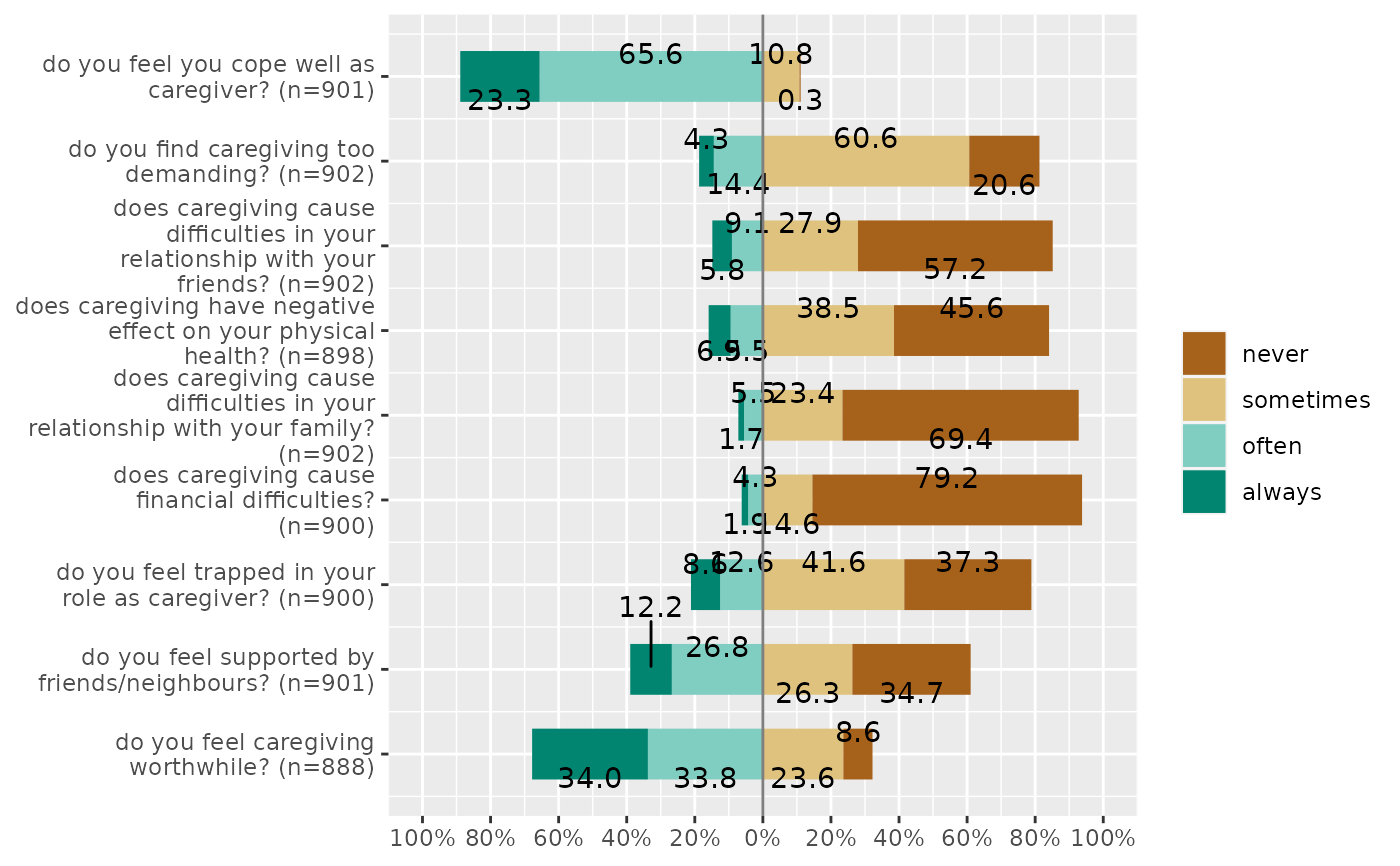plot_likert(
mydf,
grid.range = c(1.2, 1.4),
expand.grid = FALSE,
values = "sum.outside",
show.prc.sign = TRUE
)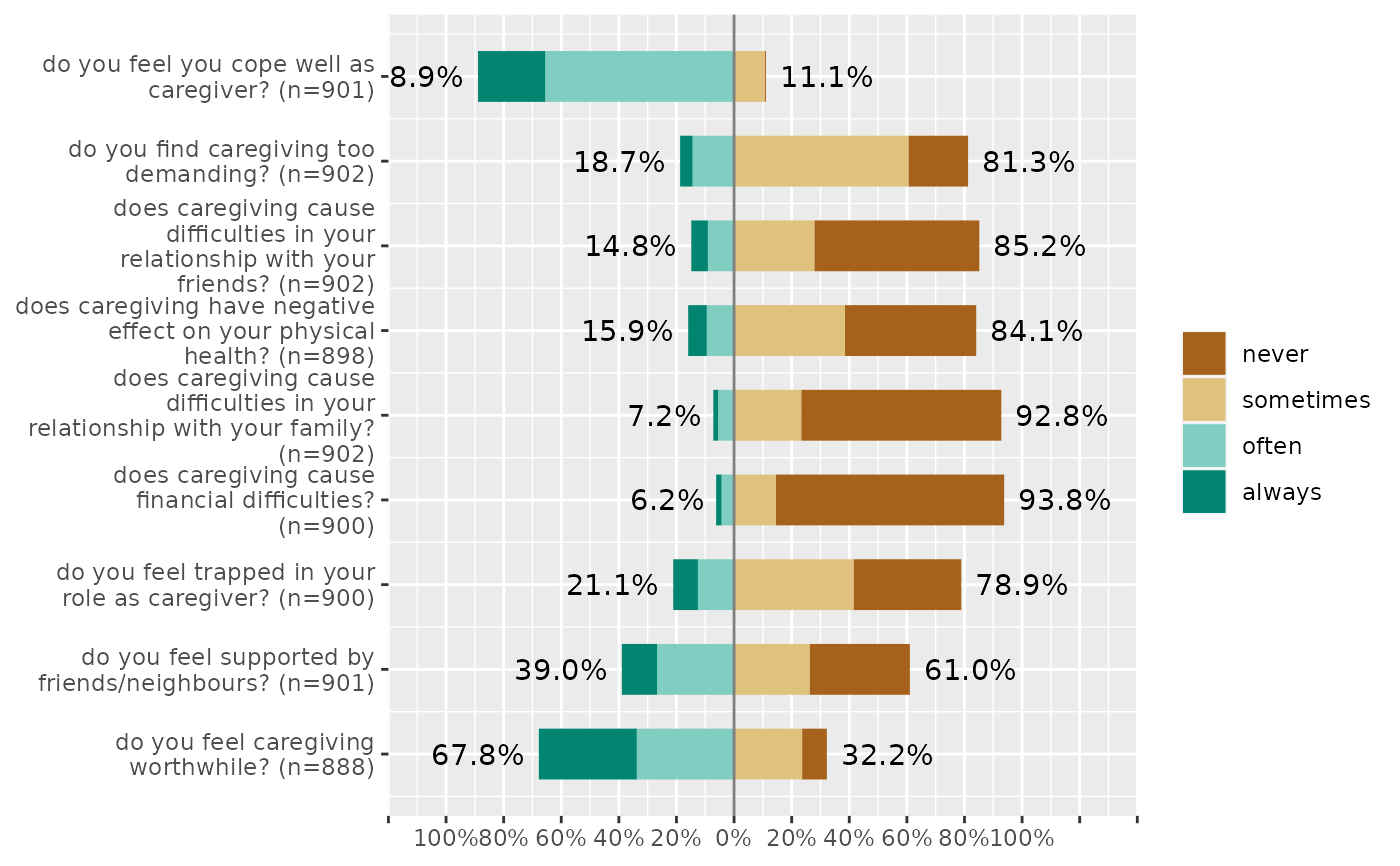# Plot in groups

plot_likert(mydf, c(2,1,1,1,1,2,2,2,1))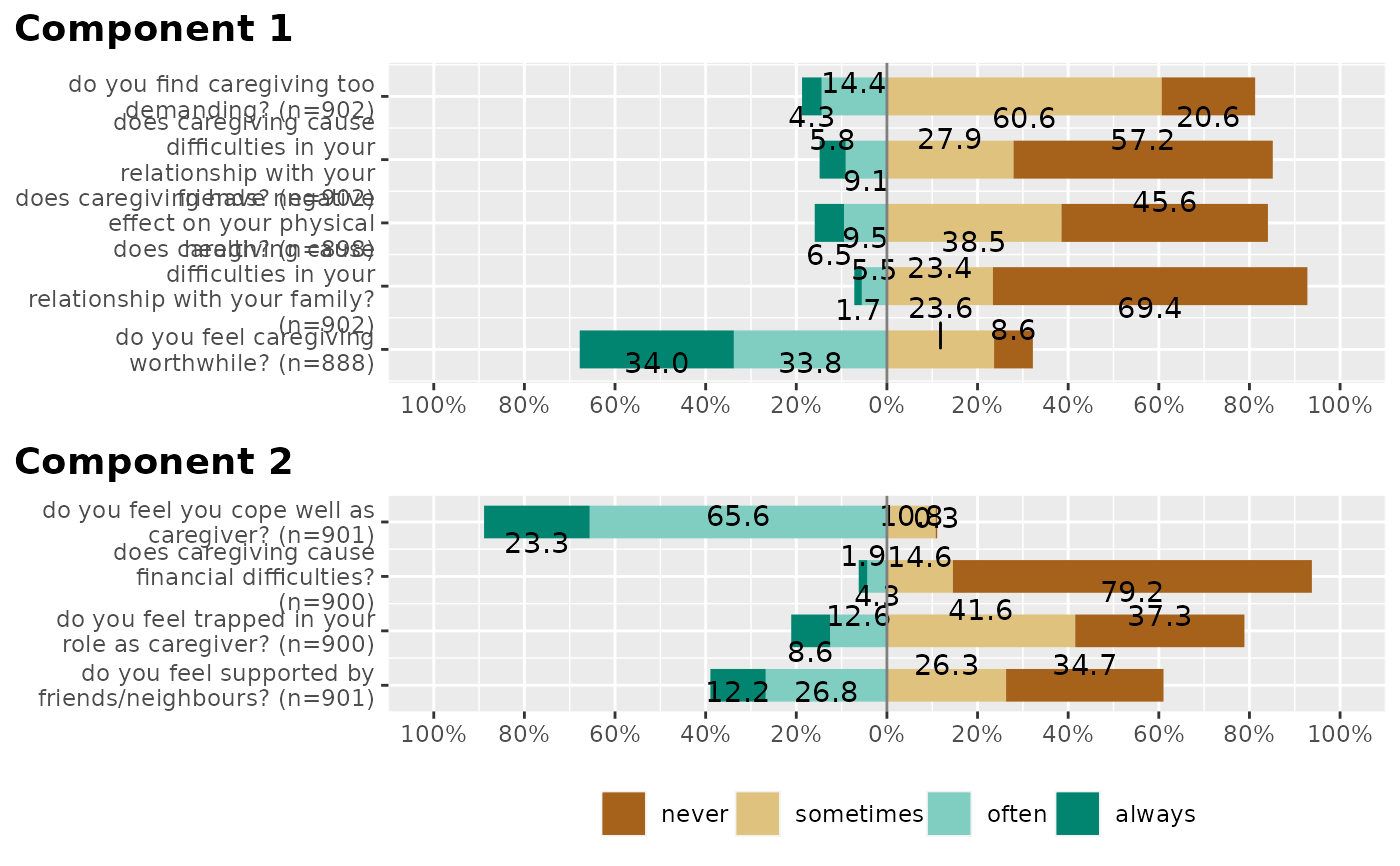if (require("parameters") && require("nFactors")) {
groups <- parameters::principal_components(mydf)
plot_likert(mydf, groups = parameters::closest_component(groups))
}
#>
#> Attaching package: ‘parameters’
#> The following object is masked from ‘package:sjmisc’:
#>
#>     center
#>
#> Attaching package: ‘nFactors’
#> The following object is masked from ‘package:lattice’:
#>
#>     parallel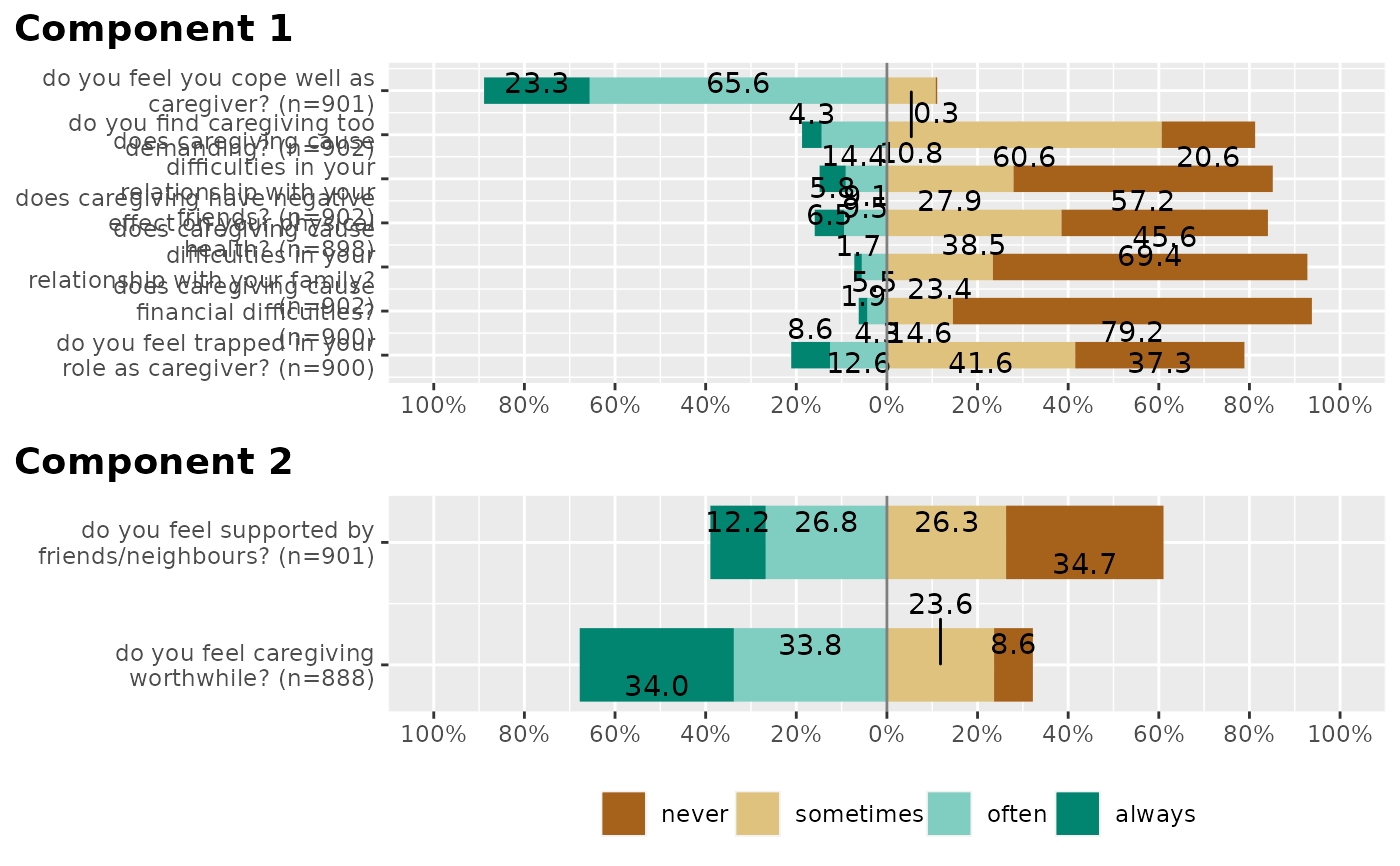plot_likert(mydf,
c(rep("B", 4), rep("A", 5)),
sort.groups = FALSE,
grid.range = c(0.9, 1.1),
geom.colors = "RdBu",
rel_heights = c(6, 8),
wrap.labels = 40,
reverse.scale = TRUE)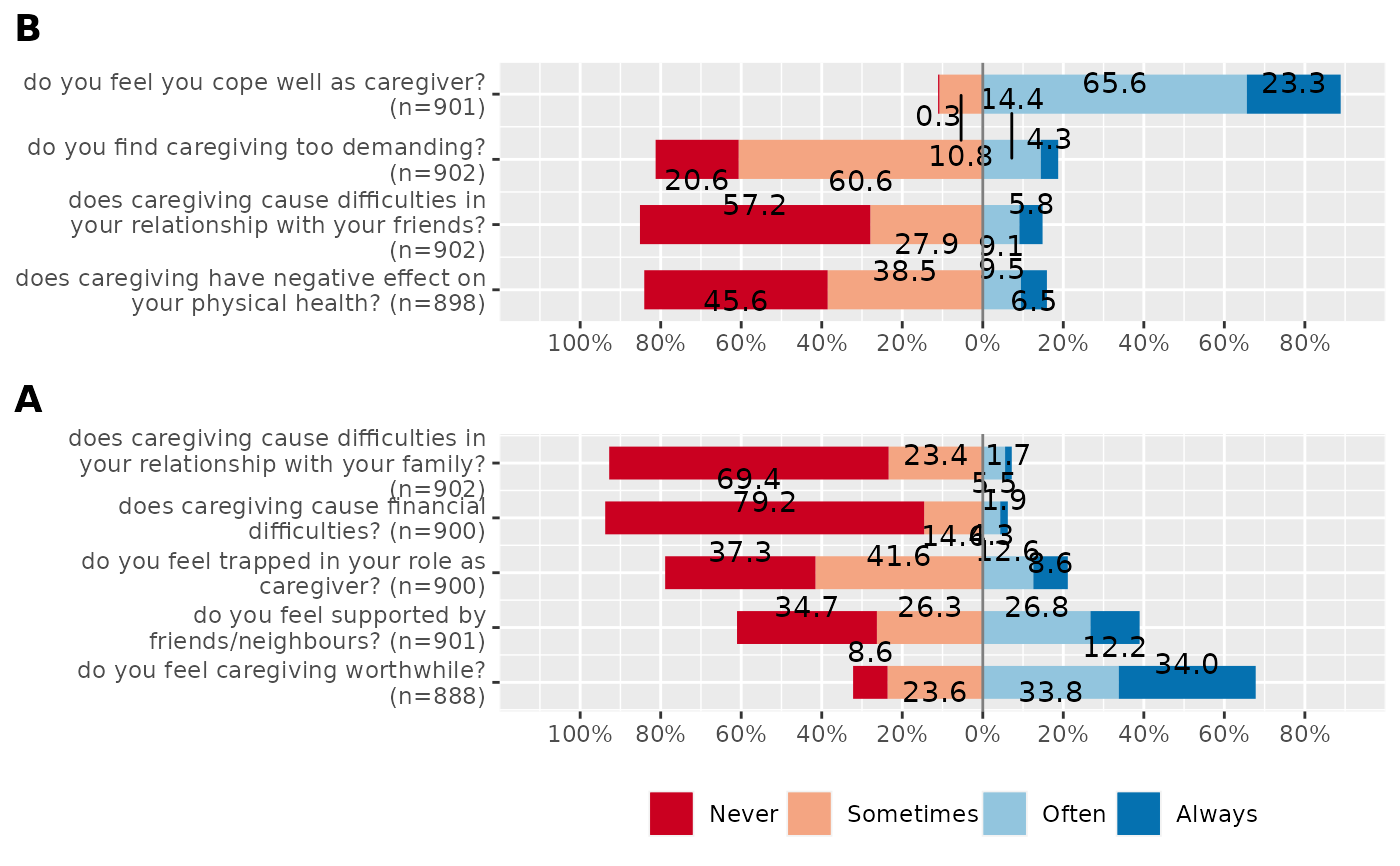# control legend items
six_cat_example = data.frame(
matrix(sample(1:6, 600, replace = TRUE),
ncol = 6)
)

if (FALSE) {
six_cat_example <-
six_cat_example %>%
dplyr::mutate_all(~ordered(.,labels = c("+++","++","+","-","--","---")))

# Old default
plot_likert(
six_cat_example,
groups = c(1, 1, 1, 2, 2, 2),
group.legend.options = list(nrow = 2, byrow = FALSE)
)

# New default
plot_likert(six_cat_example, groups = c(1, 1, 1, 2, 2, 2))

# Single row
plot_likert(
six_cat_example,
groups = c(1, 1, 1, 2, 2, 2),
group.legend.options = list(nrow = 1)
)}